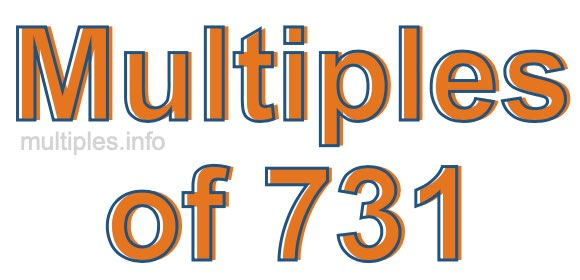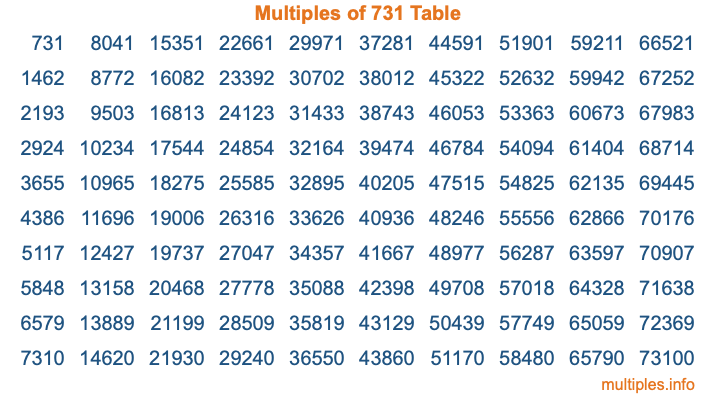Multiples of 731Welcome to the Multiples of 731 page. Here we will first teach you everything you will ever need to know about the multiples of 731, and then give you a study guide summary of everything we taught you to make sure you remember it all. Use this page to look up facts and learn information about the multiples of 731. This page will make you a multiples of seven hundred thirty-one expert!

Definition of Multiples of 731
Multiples of 731 are all the numbers that when divided by 731 equal an integer. Each of the multiples of 731 are called a multiple. A multiple of 731 is created by multiplying 731 by an integer.

Therefore, to create a list of multiples of 731, you start with 1 multiplied by 731, then 2 multiplied by 731, then 3 multiplied by 731, and so on for as long as you want. Thus, the list of the first five multiples of 731 is 731, 1462, 2193, 2924, and 3655. To see a larger list of multiples of 731, see the printable image of Multiples of 731 further down on this page. We also have a category where you can choose any nth multiple of 731.

Multiples of 731 Checker
The Multiples of 731 Checker below checks to see if any number of your choice is a multiple of 731. In other words, it checks to see if there is any number (integer) that when multiplied by 731 will equal your number. To do that, we divide your number by 731. If the the quotient is an integer, then your number is a multiple of 731.

Is  a multiple of 731?

Least Common Multiple of 731 and ...
A Least Common Multiple (LCM) is the lowest multiple that two or more numbers have in common. This is also called the smallest common multiple or lowest common multiple and is useful to know when you are adding our subtracting fractions. Enter one or more numbers below (731 is already entered) to find the LCM.

Check out our LCM Calculator if you need more details about the Least Common Multiple or if you need the LCM for different numbers for adding and subtraction fractions.

nth Multiple of 731
As we stated above, 731 is the first multiple of 731, 1462 is the second multiple of 731, 2193 is the third multiple of 731, and so on. Enter a number below to find the nth multiple of 731.

th multiple of 731

Multiples of 731 vs Factors of 731
731 is a multiple of 731 and a factor of 731, but that is where the similarities end. All postive multiples of 731 are 731 or greater than 731. All positive factors of 731 are 731 or less than 731.

Below is the beginning list of multiples of 731 and the factors of 731 so you can compare:

Multiples of 731: 731, 1462, 2193, 2924, 3655, etc.

Factors of 731: 1, 17, 43, 731

As you can see, the multiples of 731 are all the numbers that you can divide by 731 to get a whole number. The factors of 731, on the other hand, are all the whole numbers that you can multiply by another whole number to get 731.

It's also interesting to note that if a number (x) is a factor of 731, then 731 will also be a multiple of that number (x).

Multiples of 731 vs Divisors of 731
The divisors of 731 are all the integers that 731 can be divided by evenly. Below is a list of the divisors of 731.

Divisors of 731: 1, 17, 43, 731

The interesting thing to note here is that if you take any multiple of 731 and divide it by a divisor of 731, you will see that the quotient is an integer.

Multiples of 731 Table
Below is an image of the first 100 multiples of 731 in a table. The table is in chronological order, column by column. The first column has the first ten multiples of 731, the second column has the next ten multiples of 731, and so on.The Multiples of 731 Table is also referred to as the 731 Times Table or Times Table of 731. You are welcome to print out our table for your studies.

Negative Multiples of 731
Although not often discussed or needed in math, it is worth mentioning that you can make a list of negative multiples of 731 by multiplying 731 by -1, then by -2, then by -3, and so on, to get the following list of negative multiples of 731:

-731, -1462, -2193, -2924, -3655, etc.

Multiples of 731 Summary
Below is a summary of important Multiples of 731 facts that we have discussed on this page. To retain the knowledge on this page, we recommend that you read through the summary and explain to yourself or a study partner why they hold true.

There are an infinite number of multiples of 731.

A multiple of 731 divided by 731 will equal a whole number.

731 divided by a factor of 731 equals a divisor of 731.

The nth multiple of 731 is n times 731.

The largest factor of 731 is equal to the first positive multiple of 731.

731 is a multiple of every factor of 731.

731 is a multiple of 731.

A multiple of 731 divided by a divisor of 731 equals an integer.

731 divided by a divisor of 731 equals a factor of 731.

Any integer times 731 will equal a multiple of 731.

Multiples of a Number
Here you can get the multiples of another number, all with the same attention to detail as we did for multiples of 731 on this page.

Multiples of
Multiples of 732
Did you find our page about multiples of seven hundred thirty-one educational? Do you want more knowledge? Check out the multiples of the next number on our list!